# GRE Subject Test: Math : Probability

## Example Questions

### Example Question #1 : Other Topics

A student has 14 piece of gum, 3 are spearmint, 5 are peppermint, and the rest are cinnamon. If one piece of gum is chosen at random, which of the following is NOT true.

The probability of picking a cinnamon is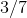.

The probability of picking a cinnamon or a peppermint is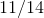.

The probability of picking a spearmint is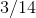.

The probability of not picking a cinnamon is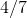.

The probability of picking a spearmint or a cinnamon is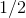.

The probability of picking a spearmint or a cinnamon is.

Explanation:

The probability of picking a spearmint or a cinnamon is the addition of probability of picking a spearmint and the probability of picking a cinnamon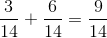not### Example Question #1 : Probability & Statistics

If I toss a coin 3 times, how many times will I roll at least one head?None of the AboveExplanation:

Step 1: We need to find out how many outcomes there will be.
If we roll a coin three times, there are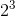outcomes.
If we roll a cointimes, there will be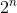outcomes.

Step 2: Find all the outcomes.

The outcomes here are: HHH, HHT, HTH, HTT, THH, THT, TTH, and TTT.

Step 3: In the list of outcomes, count how many times the letter H appears at least once.

The letter "H" appears in HHH, HHT, HTH, HTT, THH, THT, and TTH.

The letter "H" appears inof theoutcomes.

The probability of getting at least one H in the outcomes in Step 2 is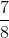.

### Example Question #1 : Probability & Statistics

How many different combinations can i have when flipping a coin three times?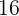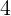Explanation:

Step 1: Let's answer a smaller problem. How many ways can I toss one coin?

There are two ways, either I get Heads or Tails.

Step 2: How about two coins?

There are four ways... They are, HH, HT, TH, and TT

Step 3: How many different combinations for three coins?
Let's List them:
HHH, HHT, HTH, THH, TTT, THT, TTH, HTT

There are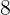different combinations.

### All GRE Subject Test: Math Resources